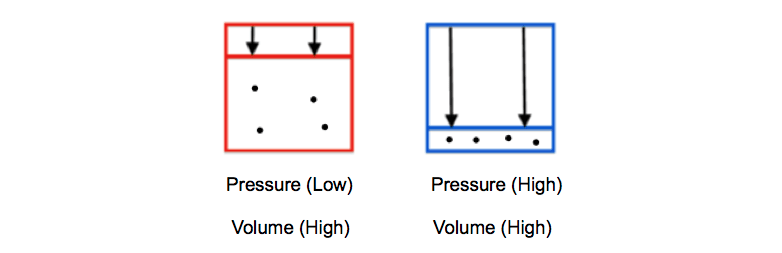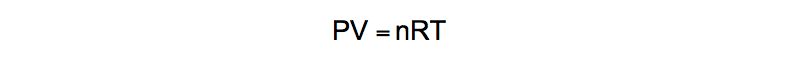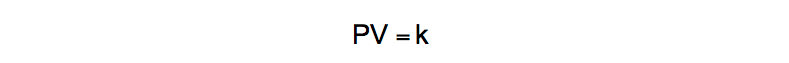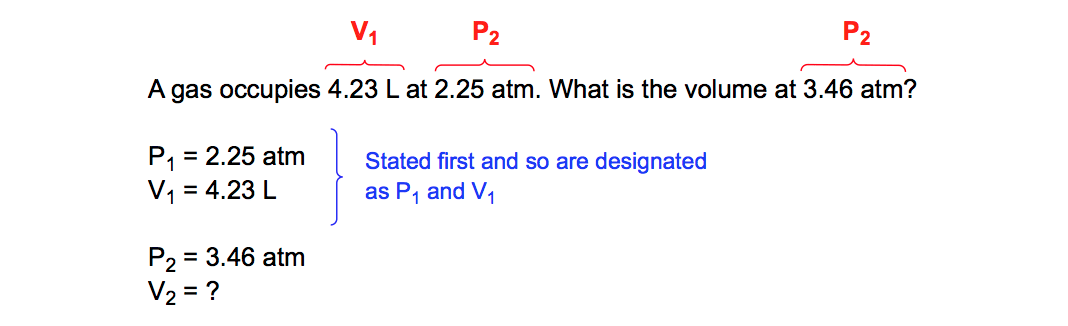Clutch Prep is now a part of Pearson

# Boyle's Law (IGNORE)

See all sections
Sections
Pressure Units
The Ideal Gas Law
The Ideal Gas Law Derivations
The Ideal Gas Law Applications
Chemistry Gas Laws
Chemistry Gas Laws: Combined Gas Law
Mole Fraction
Partial Pressure
The Ideal Gas Law: Molar Mass
The Ideal Gas Law: Density
Gas Stoichiometry
Standard Temperature and Pressure
Effusion
Root Mean Square Speed
Kinetic Energy of Gases
Maxwell-Boltzmann Distribution
Velocity Distributions
Kinetic Molecular Theory
Van der Waals Equation
Boyle's Law (IGNORE)
Charles Law (IGNORE)
Ideal Gas Law (IGNORE)Jules Bruno

Boyle’s Law, also known as Mariotte’s Law or the Boyle-Mariotte Law, states that pressure and volume are inversely proportional as long as moles (n) and temperature (T) are held constant.

Boyle’s Law

In a closed container, pressure represents the downward force applied to it while the volume represents the free space inside of it. According to Boyle’s Law, as the pressure on a container increases then the inside volume will decrease and vice-versa.Inversion Relationship of Pressure & Volume

This opposing relationship can be illustrated by the following pressure-volume graph:PV Graph

The inverse relationship between pressure and volume at a constant mass for a gas at a fixed temperature is illustrated by the expression:Boyle's Law Expression

Boyle’s Law Formula

The Ideal Gas Law is presented as:Ideal Gas Law

If moles (n) and temperature (T) are held constant then the formula simplifies into:Ideal Gas Law (Simplified)

In this form k represents our constant and when dealing with two sets of data for pressure and volume we obtain the Boyle’s Law Formula as:Boyle's Law Formula

Now let’s use it in a practice problem.

PRACTICE: A gas occupies 4.23 L at 2.25 atm. What is the volume at 3.46 atm?

STEP 1: Identify the variables given.Identifying given variables (Boyle's Law)

STEP 2: Isolate the missing variable for the second volume (V2).Solving missing variable (V2)

Beyond Boyle’s Law

Boyle’s Law represents one of the Simple Gas Laws besides Charles Law, Avogadro’s Law and Gay-Lussac’s Law. In order to understand non-ideal gases we must examine the kinetic theory of gases and the Van der Waals equationJules Bruno

Jules felt a void in his life after his English degree from Duke, so he started tutoring in 2007 and got a B.S. in Chemistry from FIU. He’s exceptionally skilled at making concepts dead simple and helping students in covalent bonds of knowledge.